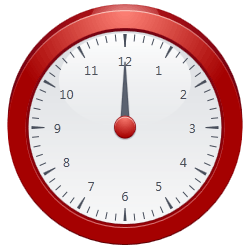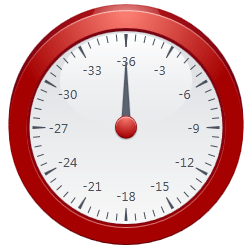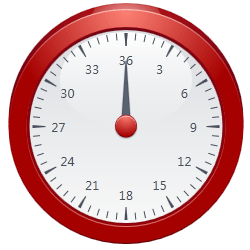# ScaleLabelOptions.Multiplier Property

Gets or sets a value by which every label’s value should be multiplied.

Namespace: DevExpress.Xpf.Gauges

Assembly: DevExpress.Xpf.Gauges.v23.1.dll

NuGet Package: DevExpress.Wpf.Gauges

## Declaration

``public double Multiplier { get; set; }``

## Property Value

Type Description
Double

A Double value which is a multiplier applied to every label’s value on a scale.

## Remarks

Use the Multiplier property, if you need to apply a multiplier to every label’s value on a scale. Note that this property affects only values displayed in scale label and doesn’t change values of any other gauge elements (e.g. value indicators).

The following images illustrate the property behavior for the Circular Scale.

Multiplier = 1 Multiplier = -3 Multiplier = 3The following code snippet (auto-collected from DevExpress Examples) contains a reference to the Multiplier property.

Note

The algorithm used to collect these code examples remains a work in progress. Accordingly, the links and snippets below may produce inaccurate results. If you encounter an issue with code examples below, please use the feedback form on this page to report the issue.